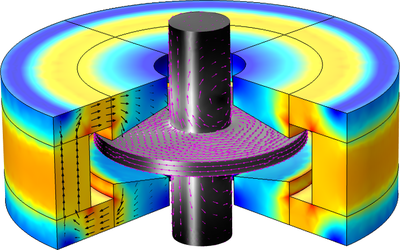# Bibliothèque d'Applications

#### Axial Homopolar Induction Bearing in 3D

Application ID: 22531

This model illustrates the working principle of an axial homopolar induction bearing. An electrically conducting rotor rotating in a magnetic field produced by a permanent magnets induces eddy currents on the conducting rotor. The eddy currents, in turn, produce a magnetic field that opposes the magnetic fields by the magnets and induces a force that opposes the motion of the rotor. The axial displacement of the rotor is always balanced by the repulsive magnetic force, thus the rotor is rotating at the center with a uniform gap. The magnetic force is calculated as a function of axial displacement.

This model is featured and explained in much greater detail in the following blog post: Modeling Magnetic Bearings in COMSOL Multiphysics.This model example illustrates applications of this type that would nominally be built using the following products: